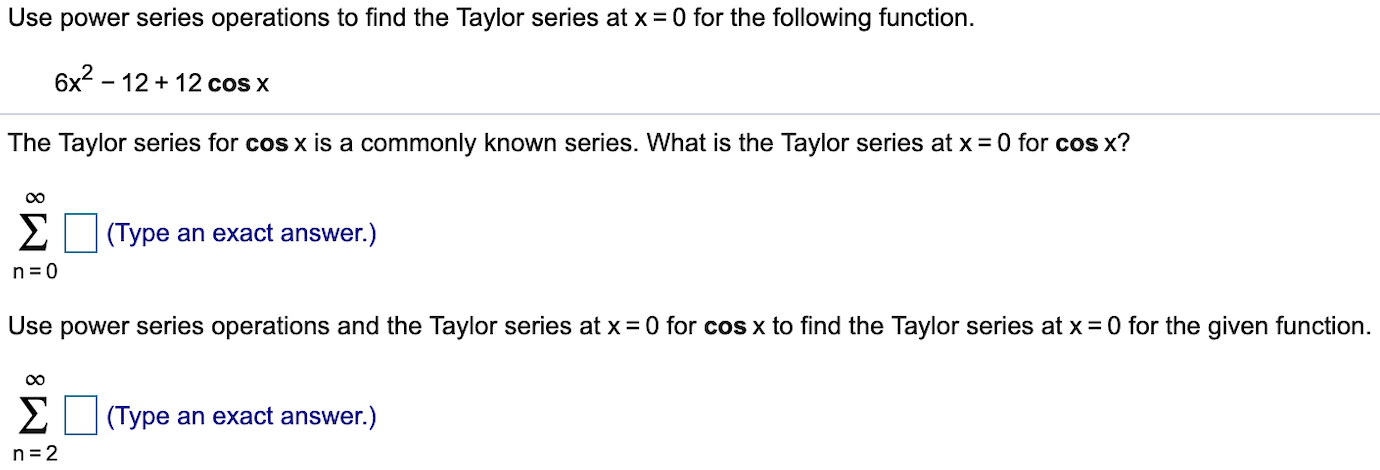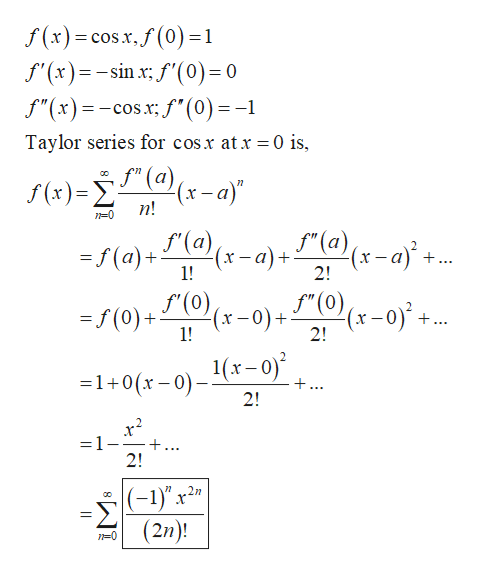Use power series operations to find the Taylor series at x 0 for the following function.6x2-1212 cos xThe Taylor series for cos x is a commonly known series. What is the Taylor series at x = 0 for cos x?Σ(Type an exact answer.)n 0Use power series operations and the Taylor series at x 0 for cos x to find the Taylor series at x 0 for the given functionΣ(Type an exact answer.)n 2

Questionhelp_outlineImage TranscriptioncloseUse power series operations to find the Taylor series at x 0 for the following function. 6x2-1212 cos x The Taylor series for cos x is a commonly known series. What is the Taylor series at x = 0 for cos x? Σ (Type an exact answer.) n 0 Use power series operations and the Taylor series at x 0 for cos x to find the Taylor series at x 0 for the given function Σ (Type an exact answer.) n 2 fullscreen
Step 1

The Taylor series for...help_outlineImage Transcriptionclosef(x) cos x,f(0) =1 f'(x)sin f'(0) = 0 _ f"(x)cos:f(0)=-1 Taylor series for cosx at x = 0 is, (x) xa)" n! n-0 - a)+ (a)(x-a +. (a)+(a) ... 1! 2! =/(0)+(x-0)+0x-0*+.. 1! 2! 1+0(x-0)-1(x-0) +... 2! =1- +. 2! (-1)" x (2n) 77 2n n-0 fullscreen

Want to see the full answer?

See Solution

Want to see this answer and more?

Our solutions are written by experts, many with advanced degrees, and available 24/7

See Solution
Tagged in

Calculus